# CUDA(六). 从并行排序方法理解并行化思维——冒泡、归并、双调排序的GPU实现

1. 充分发挥硬件能力（尽量不要有空闲且一直处于等待状态的SM）
2. 限制branch divergence（见CUDA系列学习（二））
3. 尽量保证内存集中访问（即防止不命中）

( 而我们在数据结构课上学习的sort算法往往不注意这几点。)

CUDA系列学习目录：

CUDA系列学习（一）An Introduction to GPU and CUDA

CUDA系列学习（二）CUDA memory & variables - different memory and variable types

CUDA系列学习（三）GPU设计与结构QA & coding练习

CUDA系列学习（五）GPU基础算法: Reduce, Scan, Histogram

# I. Bubble Sort

1996年， J Kornerup针对这些问题提出了odd-even sort算法，并在论文中证明了其排序正确性。

## I.1 从Bubble Sort到Odd-even Sort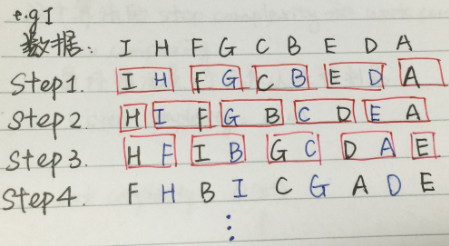PS： 对于array中有偶数个元素的数组也是一样：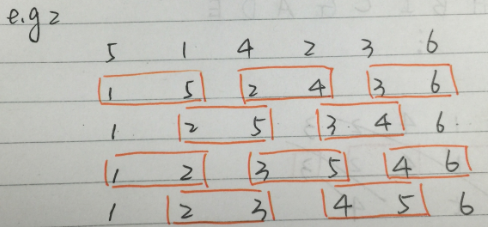## I.2 Odd-even Sort复杂度

step complexity = O(n)
work complexity = O(n^2)

code详见 < Bubble sort and its variants >

# II. Merge Sort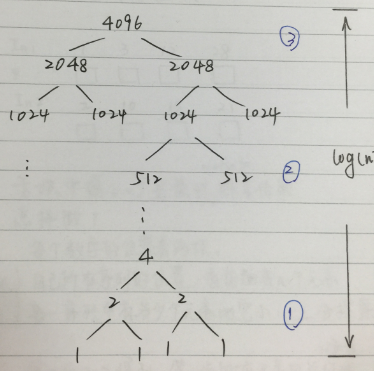## II.2 Step 2: Mid number of mid task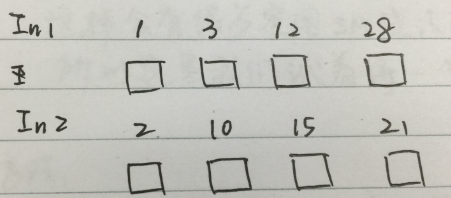1. 自己所在序列的位置： 看它前面有几个元素
2. 另一个序列的位置： 另一个序列中有多少个元素比它小（采用二分搜索）

## II.3 Step 3: Small number of huge task

1. 计算出E在In1中的位置posE1, A在In2中的位置posA2
2. merge In1中 posE1~A 和 In2中 E~posA2的元素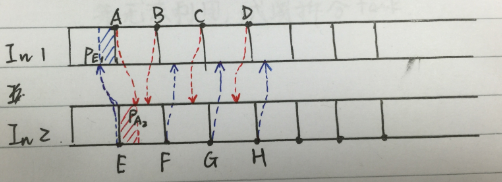## II.4 Merge sort in GPU

__global__ void mergeBlocks(int *a, int *temp, int sortedsize)
{
int id = blockIdx.x;

int index1 = id * 2 * sortedsize;
int endIndex1 = index1 + sortedsize;
int index2 = endIndex1;
int endIndex2 = index2 + sortedsize;
int targetIndex = id * 2 * sortedsize;
int done = 0;
while (!done)
{
if ((index1 == endIndex1) && (index2 < endIndex2))
temp[targetIndex++] = a[index2++];
else if ((index2 == endIndex2) && (index1 < endIndex1))
temp[targetIndex++] = a[index1++];
else if (a[index1] < a[index2])
temp[targetIndex++] = a[index1++];
else
temp[targetIndex++] = a[index2++];
if ((index1 == endIndex1) && (index2 == endIndex2))
done = 1;
}
}


        int blocks = BLOCKS/2;
while (blocks > 0)
{
mergeBlocks<<<blocks,1>>>(dev_a, dev_temp, sortedsize);
cudaMemcpy(dev_a, dev_temp, N*sizeof(int), cudaMemcpyDeviceToDevice);
blocks /= 2;
sortedsize *= 2;
}
cudaMemcpy(a, dev_a, N*sizeof(int), cudaMemcpyDeviceToHost);


# III. Bitonic Sort

## III.2 双调排序算法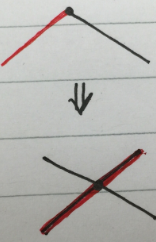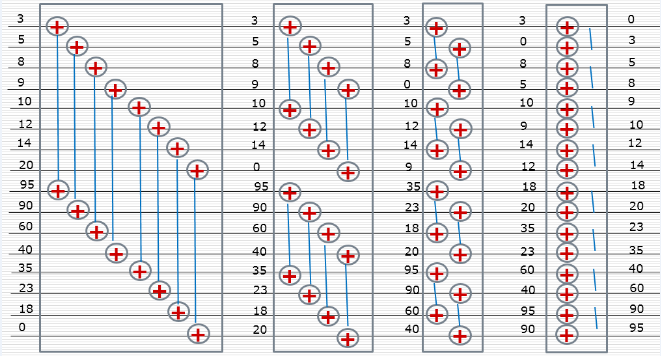## III.3 任意序列生成双调序列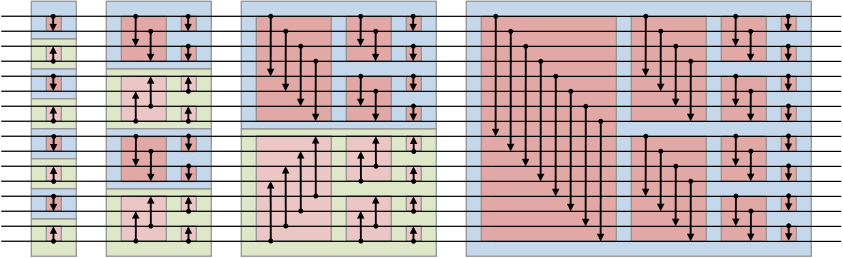1. 相邻两个元素合并形成8个单调性相反的单调序列，
2. 两两序列合并，形成4个双调序列，分别按相反单调性排序
3. 4个长度为4的相反单调性单调序列，相邻两个合并，生成两个长度为8的双调序列，分别排序
4. 2个长度为8的相反单调性单调序列，相邻两个合并，生成1个长度为16的双调序列，排序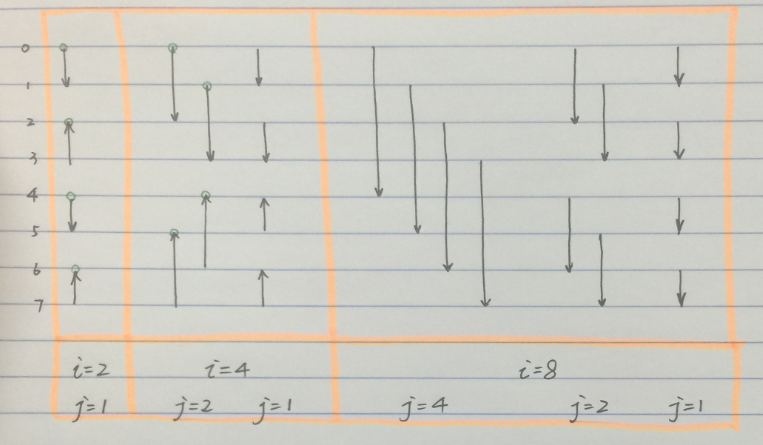## III.4 双调排序的并行实现

/*
* Author: Rachel
* <zhangruiqing01@baidu.com>
*
* File: bitonic_sort.cu
* Create Date: 2015-08-05 17:10:44
*
*/

#include<iostream>
#include<stdio.h>
#include<stdlib.h>
#include"gputimer.h"
#include<time.h>
#define NBlocks 4

__device__ void swap(int &a, int &b){
int t = a;
a = b;
b = t;
}

__global__ void bitonic_sort(int* arr){
extern __shared__ int shared_arr[];
const unsigned int tid = blockIdx.x * blockDim.x + threadIdx.x;
//const unsigned int tid = threadIdx.x;
shared_arr[tid] = arr[tid];

//for(int i=2; i<=blociDim.x; i<<=1){
for(unsigned int i=2; i<=Num; i<<=1){
for(unsigned int j=i>>1; j>0; j>>=1){
unsigned int tid_comp = tid ^ j;
if(tid_comp > tid){
if((tid & i)==0){ //ascending
if(shared_arr[tid]>shared_arr[tid_comp]){
swap(shared_arr[tid],shared_arr[tid_comp]);
}
}
else{ //desending
if(shared_arr[tid]<shared_arr[tid_comp]){
swap(shared_arr[tid],shared_arr[tid_comp]);
}
}
}
}
}
arr[tid] = shared_arr[tid];
}

int main(int argc, char* argv[])
{
GPUTimer timer;
int* arr= (int*) malloc(Num*sizeof(int));

//init array value
time_t t;
srand((unsigned)time(&t));
for(int i=0;i<Num;i++){
arr[i] = rand() % 1000;
}

//init device variable
int* ptr;
cudaMalloc((void**)&ptr,Num*sizeof(int));
cudaMemcpy(ptr,arr,Num*sizeof(int),cudaMemcpyHostToDevice);

for(int i=0;i<Num;i++){
printf("%d\t",arr[i]);
}
printf("\n");

dim3 blocks(NBlocks,1);

timer.start();
//bitonic_sort<<<1,Num,Num*sizeof(int)>>>(ptr);
timer.stop();

cudaMemcpy(arr,ptr,Num*sizeof(int),cudaMemcpyDeviceToHost);
for(int i=0;i<Num;i++){
printf("%d\t",arr[i]);
}
printf("\n");

cudaFree(ptr);
return 0;
}


code中，
tid^j用于单方向判断， 防止同一元素比较两次；
tid & i == 0 用于判断这个部分应该是单增还是单减， 因为方向在每个长为i的单调序列中是一致的， 所以选用i判断单调方向。

09-193032

#### CUDA实现QuickSortr排序算法(一)

03-091万+

#### CUDA 高性能并行计算入门12-061509

#### cuda实现奇偶冒泡排序

03-2514万+

#### 当HR压你价，说你只值7K，你该怎么回答？

03-185万+

#### 带了6个月的徒弟当了面试官，而身为高级工程师的我天天修Bug......

08-2710万+

#### 程序员求助：腾讯面试题，64匹马8个跑道，多少轮选出最快的四匹

03-1019万+

#### 副业收入是我做程序媛的3倍，工作外的B面人生是怎样的？

10-311万+

#### 【并行计算】Bitonic Sort（双调排序）基础

03-0958万+

#### 推荐 130 个令你眼前一亮的网站，总有一个用得着©️2020 CSDN 皮肤主题: 大白 设计师: CSDN官方博客点击重新获取扫码支付1.余额是钱包充值的虚拟货币，按照1:1的比例进行支付金额的抵扣。
2.余额无法直接购买下载，可以购买VIP、C币套餐、付费专栏及课程。余额充值